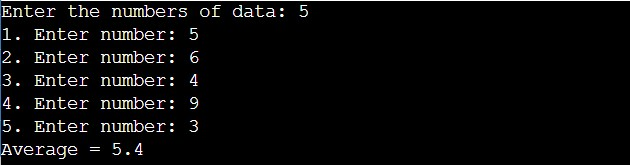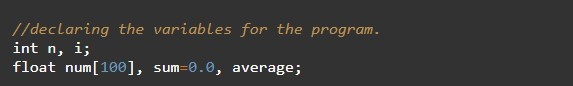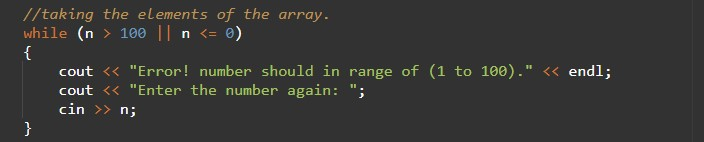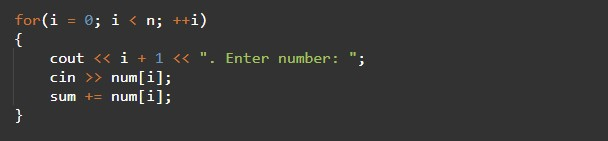# C++ Program to Calculate Average of Numbers Using Arrays

In this tutorial you will learn about the C++ Program to Calculate Average of Numbers Using Arrays and its application with practical example.

## C++ Program to Calculate Average of Numbers Using Arrays

In this tutorial, we will learn to create a C++ program that will average the elements of Array using C++ programming.

## Prerequisites

Before starting with this tutorial we assume that you are best aware of the following C++ programming topics:

• Operators in C++ Programming.
• Basic Input and Output function in C++ Programming.
• Basic C++ programming.
• For loop in C++ Programming.

## Average of numbers:-

The average number means the sum of all the terms, divided by the number of terms. The resultant will be the average of numbers.

## Program to Calculate Average of Numbers Using Arrays:-

As we all know array is a collection of similar data type elements. we can perform many different operations on arrays in c++ programming. In today’s program, we will take an array as an input and then we will find the average of all of its elements.

## Program:-

To find the Average value element from the array

## Output:-In the above program, we have first initialized the required variable• num = it will hold the elements in an array.
• n = it will hold the number of elements in an array.
• i = it will hold the integer value to control the array.
• sum= it will hold the float value for the sum of the array.
• avg= it will hold the float value for the average of the array.

Taking input from the user in an array number of elements in the array.Taking input from the user in array elements in the array.Sum of the array.Calculating the Average of Array printing the output number.In this tutorial we have learn about the C++ Program to Calculate Average of Numbers Using Arrays and its application with practical example. I hope you will like this tutorial.